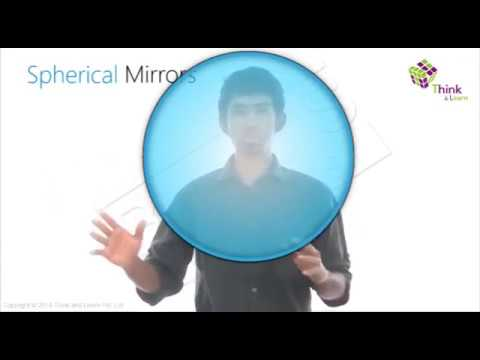# Define the Principal Focus of a Concave Mirror?

Light rays that are parallel to the principal axis of a concave mirror converge at a specific point on its principal axis after reflecting from the mirror. This point is known as the principal focus of the concave mirror.

Characteristics of the image obtained by a concave mirror
There are five cases through which one can understand the characteristics of the image obtained by a concave mirror and they are as follows:

 Object placement Image obtained Image characteristics When the object is placed at infinity The image obtained is at the focal point Image is a point When the object is moved from infinity to the centre of curvature Image move from focal point to centre of curvature The size of the image is increased, real, and inverted When the object is at the centre of curvature The image obtained is at the centre of the curvature The size of the image remains the same, real, and inverted When the object moves from centre of curvature to focal point Image move from centre of curvature to infinity The size of the image is bigger than the object, real, and inverted When the object is at the focal point The image is at infinity Infinitely big When the object moves from the focal point to mirror Image move from minus infinity to mirror The size of the image decreases, larger than the object, virtual, and upright

Check out the video given below to know more about spherical mirrorRelated Questions:

3 (20)(50)(14)

#### Choose An Option That Best Describes Your Problem

Thank you. Your Feedback will Help us Serve you better.

1. MOHD ehsaan dar

Veary nice class

• Samna khan

Very nice class

2. Anjali dhyani

To good

3. Abhinav

Wow

4. Its too good